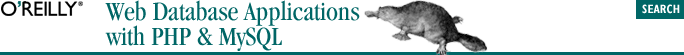home | O'Reilly's CD bookshelfs | FreeBSD | Linux | Cisco | Cisco Exam## 2.10. User-Defined Functions

#### Example 2-6. A user-defined function to output bold text

```<!DOCTYPE HTML PUBLIC
"-//W3C//DTD HTML 4.0 Transitional//EN"
"http://www.w3.org/TR/html4/loose.dtd">
<html>
<title>Simple Function Call</title>
<body bgcolor="#ffffff">
<?php

function bold(\$string)
{
echo "<b>" . \$string . "</b>\n";
}

// First example function call (with a static string)
echo "this is not bold\n";
bold("this is bold");
echo "this is again not bold\n";

// Second example function call (with a variable)
\$myString = "this is bold";
bold(\$myString);
?>
</body></html>```
```function heading(\$text, \$headingLevel)
{
case 1:
\$result = "<h1>" . ucwords(\$text) . "</h1>";
break;

case 2:
\$result = "<h2>" . ucwords(\$text) . "</h2>";
break;

case 3:
\$result = "<h3>" . ucfirst(\$text) . "</h3>";
break;

default:
\$result = "<p><b>" . ucfirst(\$text) . "</b>";

return(\$result);
}

\$test = "user defined functions";

The function takes two parameters: the text of a heading and a heading level. Based on the value of \$headingLevel, the function builds the HTML suitable to display the heading—changing the case of the \$text appropriately. The previous fragment generates the string:

`<h2>User Defined Functions</h2>`

The variable that is returned by a return statement can optionally be placed in parentheses: the statements return(\$result) and return \$result are identical.

### 2.10.2. Variable Scope

```function doublevalue(\$var)
{
\$temp = \$var * 2;
}

\$variable = 5;
doublevalue(\$variable);
echo "\\$temp is: \$temp";```

This example outputs the string:

`\$temp is:`

If you want to use a value that is local to a function elsewhere in a script, the easiest way to do so is to return the value of the variable. This example achieves this:

```function doublevalue(\$var)
{
\$returnVar = \$var * 2;
return(\$returnVar);
}

\$variable = 5;
\$temp = doublevalue(\$variable);
echo "\\$temp is: \$temp";```

The example prints:

`\$temp is: 10`

You could have still used the variable name \$temp inside the function doublevalue( ). However, the \$temp inside the function is a different variable from the \$temp outside the function. The general rule is that variables used exclusively within functions are local to the function, regardless of whether an identically named variable is used elsewhere. There are two exceptions to this general rule: variables passed by reference and those declared global in the function aren't local to the function.